# OECD Business confidence Index(BCI) data analysis 6 - There is no monthly/quarterly seasonality for BCI.Photo by Juliane Liebermann on Unsplash

This post is following of above post.

Now, let's see df dataframe object again.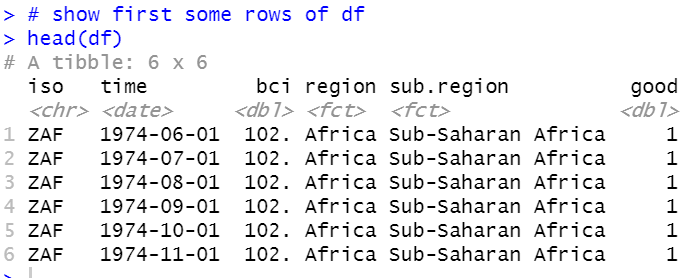df have "time" variable as Date class. I will make year and month from time.
I need lubridate package.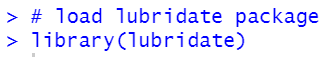Then, I use year() function and month(function).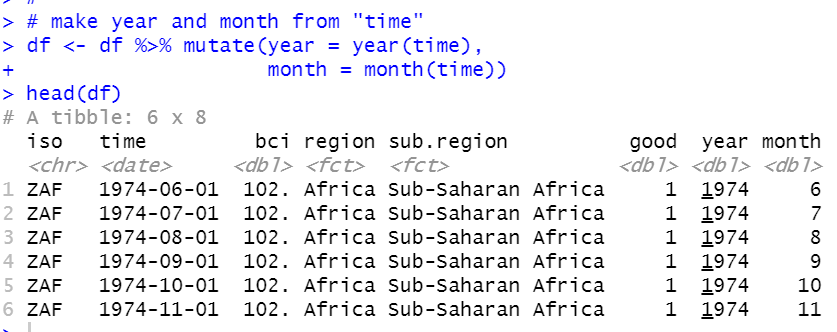Let's calculate bci summary statistics by year.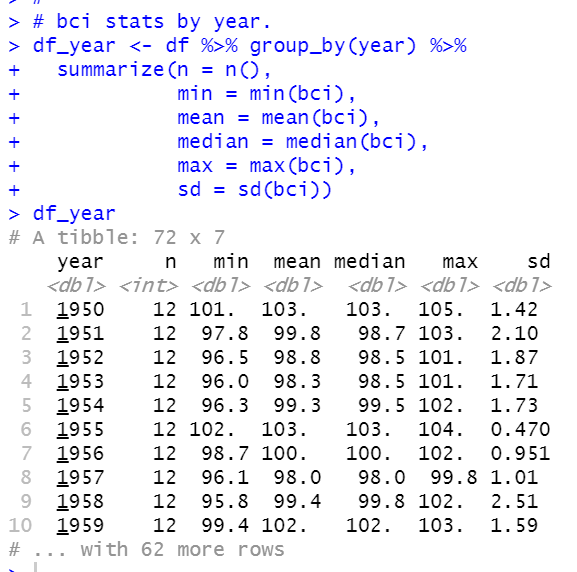Let's plot those data.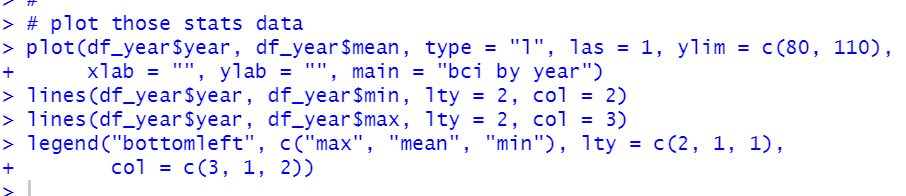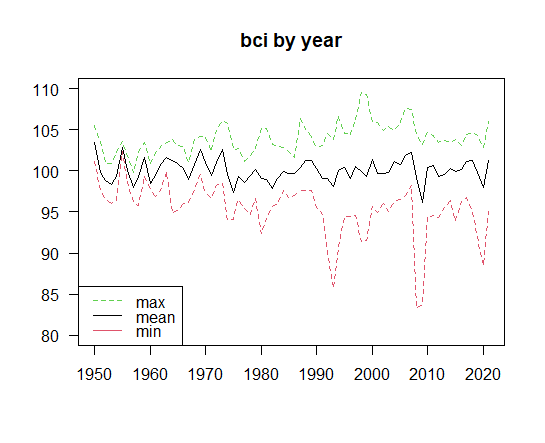Let's calculate summary statistics by month.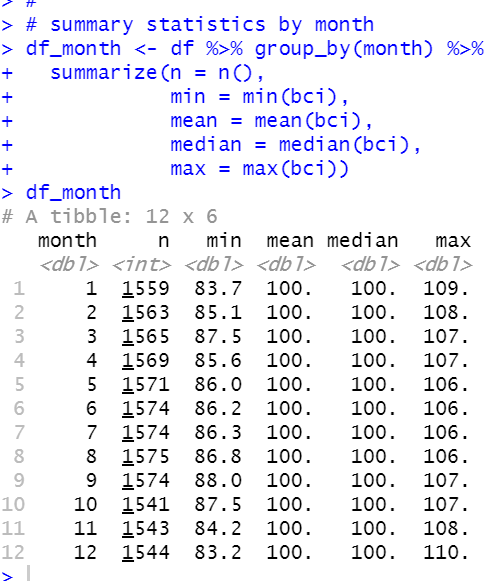I would like to mode decimal places for mean and median.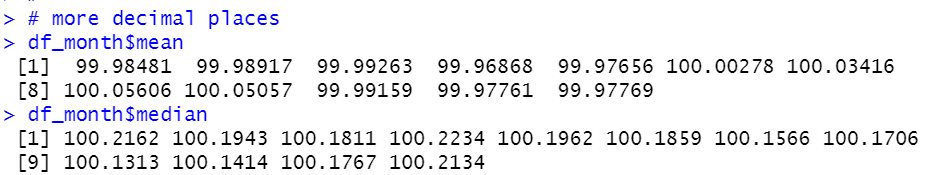For mean, June, July, August and September are above 100.

Let's do ANOVA analysis to check if there is significant differnece by month.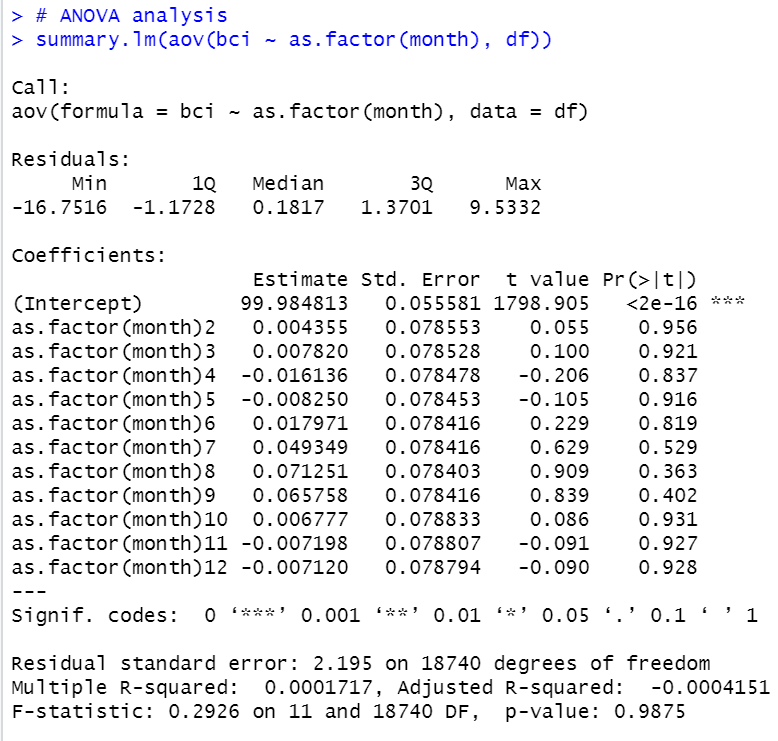p-value is 0.9875. So we cannot reject null hypothese. null is "there is significant differnece by month.".

January, February and March is the 1st Quarter,

April, May and June is the 2nd Quarter,

July, August and  September is the 3rd Quarter,

October, November and December is 4th Quarter.

I use case_when() function to make quarter variable.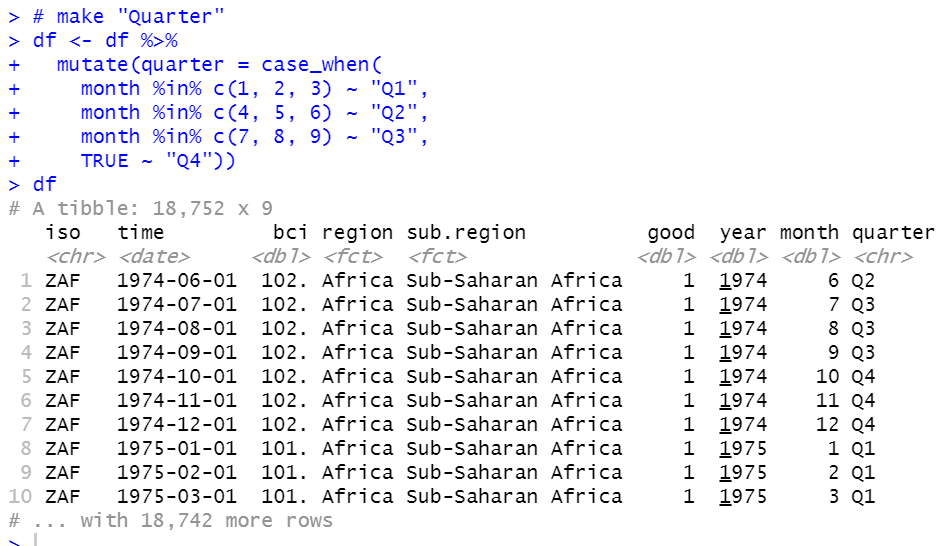Let's do ANOVA by quarter.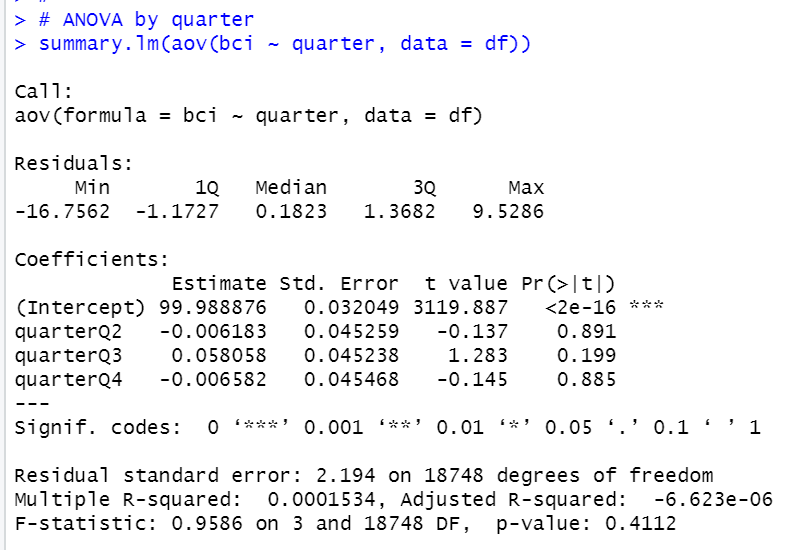p-value is 0.4112. So there is no quarterly seasonality for BCI.

That's all. Thank you!

Next post is

To read from the 1st post,